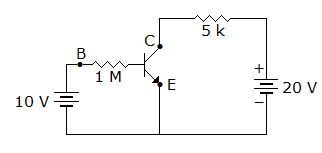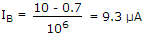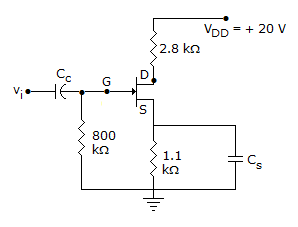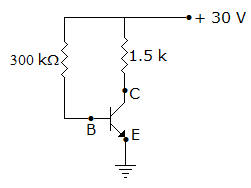# Electronics and Communication Engineering - Analog Electronics

26.

Maximum efficiency of class B power amplifier is 50%.

 A. True B. False

Explanation:

Maximum efficiency for class B-operation can be 78.5% .

27.

In figure what is the base current if VBE = 0.7 VA. 10 mA B. 9.3 mA C. 10 μA D. 9.3 μA

Explanation:.

28.

In figure ID = 4 mA. Then VS =A. 20 V B. 4.4 V C. 0.4 V D. 0.44 V

Explanation:

VS = ISRS = 4 x 10-3 x 1.1 x 103 = 4.4 V.

29.

The self bias provides

 A. stable Q point B. large voltage gain C. high input impedance D. high base current

Explanation:

Stable Q point is the advantage of self bias circuit.

30.

In figure what is value of IC if βdc = 100. Neglect VBEA. 5 mA B. 5 μA C. 10 mA D. 10 μA